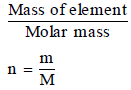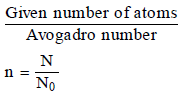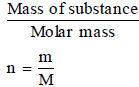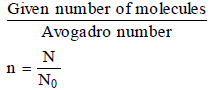Courses

# Atoms and Molecules, Chapter Notes, Class 9, Science Class 9 Notes | EduRev

Created by: Learners Habitat

## Class 9 : Atoms and Molecules, Chapter Notes, Class 9, Science Class 9 Notes | EduRev

The document Atoms and Molecules, Chapter Notes, Class 9, Science Class 9 Notes | EduRev is a part of Class 9 category.
All you need of Class 9 at this link: Class 9

While doing numerical problems of mole concept, the following relations should be remembered.

Key for symbols

Number of moles = n

Given mass = m

Molar mass = M

Given number of particle = N

Avogadro number of particle = N0

Key Formula

1 mole of atoms = 6.022 × 1023 atoms = Gram atomic mass or Molar mass of element

Number of moles =Number of moles =These relations can be interchanged as

Mass of element, m = n × M

or Number of particles of element, N = n × N0

Similarly,

1 mole of molecules = 6.022 × 1023 molecules = Gram molecular mass or Molar mass

Number of moles =Number of moles =or m = n × M and N = n × N0

Let us apply these formula to solve numerical problems.

,

,

,

,

,

,

,

,

,

,

,

,

,

,

,

,

,

,

,

,

,

,

,

,

,

,

,

,

,

,

;